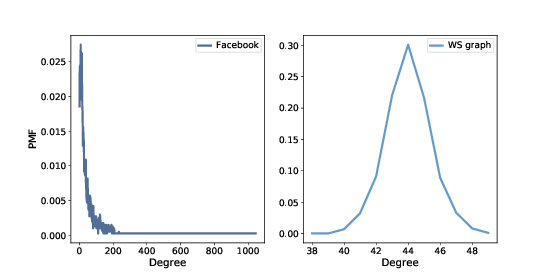# 6.4. Degree¶Figure 6.1: PMF of degree in the Facebook dataset and in the WS model.

If the WS graph is a good model for the Facebook network, it should have the same average degree across nodes, and ideally the same variance in degree.

This function returns a list of degrees in a graph, one for each node:

def degrees(G):
return [G.degree(u) for u in G]


The mean degree in model is $$44$$, which is close to the mean degree in the dataset, $$43.7$$.

However, the standard deviation of degree in the model is $$1.5$$, which is not close to the standard deviation in the dataset, $$52.4$$. Oops.

What’s the problem? To get a better view, we have to look at the distribution of degrees, not just the mean and standard deviation.

We will represent the distribution of degrees with a Pmf object, which is defined in the thinkstats2 module. Pmf stands for “probability mass function”.

Briefly, a Pmf maps from values to their probabilities. A Pmf of degrees is a mapping from each possible degree, d, to the fraction of nodes with degree d.

As an example, we construct a graph with nodes $$1$$, $$2$$, and $$3$$ connected to a central node, $$0$$:

G = nx.Graph()
nx.draw(G)


Here’s the list of degrees in this graph:

>>> degrees(G)
[3, 1, 1, 1]


Node $$0$$ has degree $$3$$, the others have degree $$1$$. Now we can make a Pmf that represents this degree distribution:

>>> from thinkstats2 import Pmf
>>> Pmf(degrees(G))
Pmf({1: 0.75, 3: 0.25})


The result is a Pmf object that maps from each degree to a fraction or probability. In this example, $$75%$$ of the nodes have degree $$1$$ and $$25%$$ have degree $$3$$.

Now we can make a Pmf that contains node degrees from the dataset, and compute the mean and standard deviation:

>>> from thinkstats2 import Pmf
>>> pmf_fb = Pmf(degrees(fb))
>>> pmf_fb.Mean(), pmf_fb.Std()
(43.691, 52.414)


And the same for the WS model:

>>> pmf_ws = Pmf(degrees(ws))
>>> pmf_ws.mean(), pmf_ws.std()
(44.000, 1.465)


We can use the thinkplot module to plot the results:

thinkplot.Pdf(pmf_fb, label='Facebook')
thinkplot.Pdf(pmf_ws, label='WS graph')


Figure 6.1 shows the two distributions. They are very different.

In the WS model, most users have about $$44$$ friends; the minimum is $$38$$ and the maximum is $$50$$. That’s not much variation. In the dataset, there are many users with only $$1$$ or $$2$$ friends, but one has more than $$1000$$!

Distributions like this, with many small values and a few very large values, are called heavy-tailed.